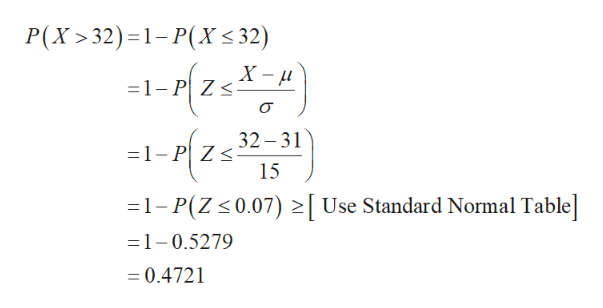# Los Angeles workers have an average commute of 31 minutes. Suppose the LA commute time is normally distributed with a standard deviation of 15 minutes. Let X represent the commute time for a randomly selected LA worker.a. What is the distribution of X? X ~ N(,)b. Find the probability that a randomly selected LA worker has a commute that is longer than 32 minutes. Round to 4 decimal places. c. Find the 80th percentile for the commute time of LA workers.  Round to 2 decimal places.  minutes

Question
5 views

Los Angeles workers have an average commute of 31 minutes. Suppose the LA commute time is normally distributed with a standard deviation of 15 minutes. Let X represent the commute time for a randomly selected LA worker.

a. What is the distribution of X? X ~ N(,)

b. Find the probability that a randomly selected LA worker has a commute that is longer than 32 minutes. Round to 4 decimal places.

c. Find the 80th percentile for the commute time of LA workers.  Round to 2 decimal places.  minutes

check_circle

Step 1

(a)

The distribution X follows normal with mean µ and standard deviation σ. That is, X~N (µ, σ).

Here, the value of mean is 31 minutes and the standard deviation is 15.

Hence, the distribution X follows normal with mean 31 (µ) and standard deviation 15 (σ). That is, X~N (31,15).

(b)

The probability that a randomly selected LA worker has a commute that is longer than 32 minutes is,help_outlineImage TranscriptioncloseP(X>32) =1-P(X< 32) -1-1 25X- Z < 32 31 -1-P Zs 15 1-P(Z 0.07) Use Standard Normal Table =1-0.5279 = 0.4721 fullscreen
Step 2

Thus, the probability that a randomly selected LA worker has a commute that is longer than 32 minutes is ...

### Want to see the full answer?

See Solution

#### Want to see this answer and more?

Solutions are written by subject experts who are available 24/7. Questions are typically answered within 1 hour.*

See Solution
*Response times may vary by subject and question.
Tagged in

### Measures